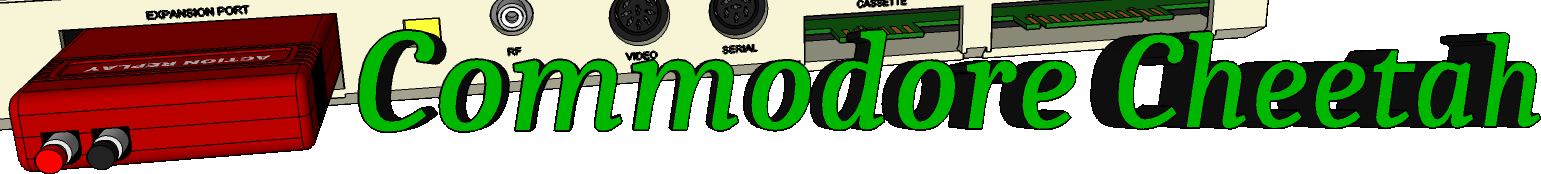Home PageeMail
email5-commodorecheetah.co.uk

Note: You will need to replace the '-' with the @ symbol.
This is to minimize spam.

My BASIC Quick Reference Guide

AND
Abbreviation: A <SHIFT+N>

TYPE: Operator
FORMAT: <expression> AND <expression>

Action: AND is used in Boolean operations to test bits. it is also used in operations to check the truth of both operands.

In Boolean algebra, the result of an AND operation is 1 only if both numbers being ANDed are 1. The result is 0 if either or both is 0 (false).

EXAMPLES of 1-Bit AND operation:

 0 AND 0 ------------ 0 1 AND 0 ------------ 0 0 AND 1 ------------ 0 1 AND 1 ------------ 1

The Commodore 64 performs the AND operation on numbers in the range from -32768 to +32767. Any fractional values are not used, and numbers beyond the range will cause an ?ILLEGAL QUANTITY error message. When converted to binary format, the range allowed yields 16 bits for each number. Corresponding bits are ANDed together, forming a 16-bit result in the same range.

EXAMPLES of 16-Bit AND Operation:

 17 AND 194 -------------------------------------------- 0000000000010001 AND 0000000011000010 -------------------------------------------- (BINARY) 0000000000000000 -------------------------------------------- (DECIMAL) 0   32007 AND 28761 -------------------------------------------- 0111110100000111 AND 0111000001011001 -------------------------------------------- (BINARY) 0111000000000001 -------------------------------------------- (DECIMAL) 28673   -241 AND 15359 -------------------------------------------- 1111111100001111 AND 0011101111111111 -------------------------------------------- (BINARY) 0011101100001111 -------------------------------------------- (DECIMAL) 15119

When evaluating a number for truth or falsehood, the computer assumes the number is true as long as its value isn't 0. When evaluating a comparison, it assigns a value of -I if the result is true, while false has a value of 0. In binary format, -1 is all 1's and 0 is all 0's. Therefore, when ANDing true/false evaluations, the result will be true if any bits in the result are true.

EXAMPLES of Using AND with True/False Evaluations:

50 IF X=7 AND W=3 THEN GOTO 10: REM ONLY TRUE IF BOTH X=7 AND W=3 ARE TRUE
60 IF A AND Q=7 THEN GOTO 10: REM TRUE IF A IS NON-ZERO AND Q=7 IS TRUE

Commodore Cheetah made by Allen Monks, started in the year 2000.